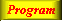Lecture 3 - Page 31 : 42
 Functional Programming in SchemeName binding, Recursion, Iteration, and Continuations * Name binding constructs The let name binding expression The equivalent meaning of let Examples with let name binding The let* name binding construct An example with let* The letrec namebinding construct LAML time functions * Conditional expressions Conditional expressions Examples with if Example with cond: leap-year? Example with cond: american-time Example with cond: as-string * Recursion and iteration Recursion List processing Tree processing (1) Tree processing (2) Recursion versus iteration Example of recursion: number-interval Examples of recursion: string-merge Examples with recursion: string-of-char-list? Exercises * Example of recursion: Hilbert Curves Hilbert Curves Building Hilbert Curves of order 1 Building Hilbert Curves of order 2 Building Hilbert Curves of order 3 Building Hilbert Curves of order 4 A program making Hilbert Curves * Continuations Introduction and motivation The catch and throw idea A catch and throw example The intuition behind continuations Being more precise The capturing of continuations Capturing, storing, and applying continuations Use of continuations for escaping purposes Practical example: Length of an improper list Practical example: Searching a binary tree
 A program making Hilbert Curves Given our understanding of Hilbert Curves obtained from the previous pages, we will now study a computer program that generates Hilbert Curves of order n, where n is any non-negative number.
 We will here discuss a concrete program which draws Hilbert Curves of order nThe function hilbert programmed in Scheme as a functional program.

The function returns the path of the Hilbert Curver of order n. The parameter turn determines the rotation of the curve. In the top level call we ask for an upward Hilbert Curve: As an example, (hilbert 3 'up) produces an upward Hilbert Curve of order 3. The red fragments are responsible for all line drawing. The blue fragments represent all the recursive calls of the hilbert function. Finally, the green fragment represent the level 0 'basis' case. The level 0 case returns the empty Hilbert Curve, which is literally empty (no drawing at all - no contribution to the resulting path). What does it mean that the the program is a functional program? Well, it basically means that hilbert returns a value which can be rendered somehow by another function or procedure. The value returned is a path, composed by concat-path. The hilbert function does not carry out any action itself.The complete Hilbert programs including SVG details.A simple Scheme path library developed for SVG.